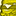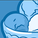Announcements
Applications are open for the 2024 Qlik Luminary Program. Apply by December 15 here.
cancel
Showing results for
Did you mean:Contributor II

## Using greater than for date variable in set expression

Im trying to calculate the 12 week rolling average prior to the most current week

The most recent date is stored in variable \$(vMaxCal) as an integer (currently it is 44365 for 6/18/2021)

To get the 12 week average I need to get date field (timeofarrival) to be (> vMaxCal - 12 weeks) essentially

how can I fix this set expression so that it works :
=sum({< [Publications.timeofarrival]={">date(\$(vMaxCal)-(7*12),'M/D/YYYY')"}, [_thisWeek]-={1}>} Publications.counter) /12

Btw, if i hard code the date in like this, it works:
=sum({< [Publications.timeofarrival]={">3/26/2021"}, [_thisWeek]-={1}>} Publications.counter) /12

also to add, the equation itself doesnt have any problem in terms of error messages, but the result in the visualization is a value of 0 instead of the actual average>

If i create a KPI with JUST date(\$(vMaxCal)-(7*12),'M/D/YYYY'), it works

1 Solution

Accepted SolutionsSpecialist

You need to enclose the date calculation in a \$-expansion, like this:

=sum({< [Publications.timeofarrival]={">\$(=date(\$(vMaxCal)-(7*12),'M/D/YYYY'))"}, [_thisWeek]-={1}>} Publications.counter) /12

2 RepliesSpecialist

You need to enclose the date calculation in a \$-expansion, like this:

=sum({< [Publications.timeofarrival]={">\$(=date(\$(vMaxCal)-(7*12),'M/D/YYYY'))"}, [_thisWeek]-={1}>} Publications.counter) /12Contributor II
Author

Ahh perfect, thank you Gary!Tags
Community Browser